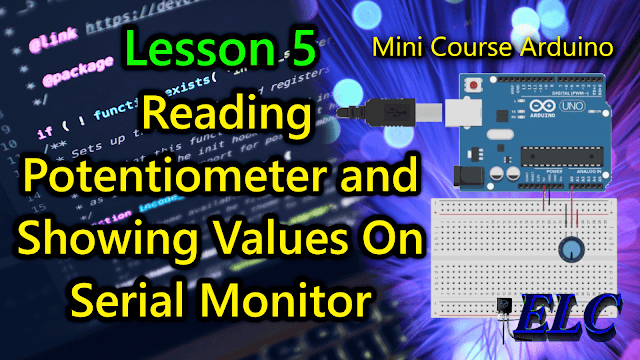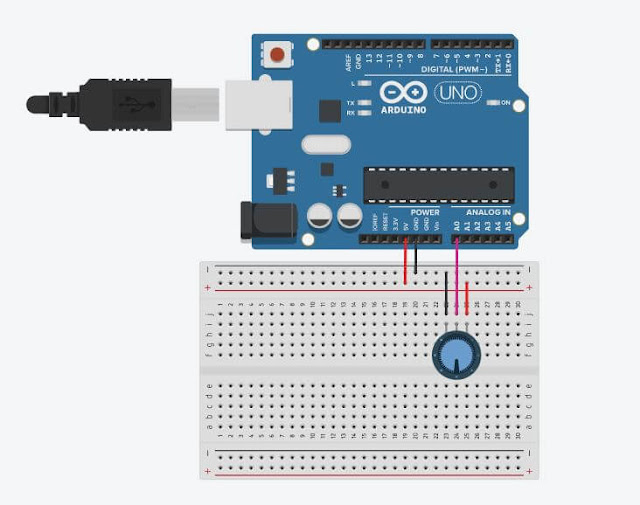## Sunday, September 19, 2021Fig. 1 - Arduino Lesson 5 - Reading Potentiometer and Showing Values On Serial Monitor

# Welcome to Lesson 5 - Basic Arduino Course

Today we are going to learn how to read a Potentiometer end showing the Values on Serial Monitor.
In this example, we will use a potentiometer, however, this same concept is used for most analog sensors.

What will differentiate will be the type of calculation used, with reference to each sensor.

We will use the analogRead() function, to read A0 PIN, in Arduino we have 6 Analog Ports, which goes from A0 to A5, so we can make readings from up to 6 sensors simultaneously without having to use external hardware.

## Hardware Required

• Arduino Board
• A 10K Potentiometer
• Wires
• Protoboard (optional)

## The Circuit

The potentiometer works like a voltage divider. When you turn the shaft of the potentiometer, you change the resistance on either side of the center pin (or wiper) of the potentiometer.

This changes the relative resistances between the center pin and the two outer pins, so you get a different voltage at the analog input.

When the shaft is fully rotated in one direction, there is no resistance between the center pin and the pin connected to ground.
The middle pin varies from 0Kohms to 10Kohms, which is the value of the potentiometer we will use.

This resistance is sensed by the voltages at the ends of the potentiometer, causing the center pin to vary between 0V and 5V

And the voltage variation that comes from the Center Pin of the potentiometer goes to the A0 Analog Port.

In Figure 2, you can see the schematic of the potentiometer and the Arduino. We use a breadboard to make the connections easier, but you can also connect the wires directly from the A0 terminal in the middle of the potentiometer, connecting the outer pin to 5V positive and the outer pin to the negative GND of the Arduino.Fig. 2 - Reading Potentiometer and Showing Values On Serial Monitor - tinkercad.com

## The Code

The analogRead() function converts the input voltage range, 0 to 5 volts, to a digital value between 0 and 1023. This is done by a circuit within the microcontroller called analog-to-digital converter, or ADC.

Then, if the voltage at the center pin is 0 volts, analogRead() returns 0. When the shaft is turned all the way the other way, there is no resistance between the middle pin and the pin connected to 5 volts

If the voltage on the middle pin is then 5 voltsanalogRead() returns 1023. In between, analogRead() returns a number between 0 and 1023 that is proportional to the voltage applied to the pin.

After building the circuit, connect your Arduino board to your computer, launch Arduino Software (IDE), copy the code below and paste it into your Arduino IDE. But first let us understand the code line by line.

• In Line 3, we declared variable sensorPin which is set to Analog Pin A0 where we receive the value of the connected potentiometer.
• In Line 4, we create a variable senorPin that stores the value of the sensor that we also use for the potentiometer.

 0102030405 // Arduino: Lesson 5 -  Reading Potentiometer and Showing Values On Serial Monitorint sensorPin = A0;            // Read the Analogic Pin A0 Values  into a variableint sensorValue = 0;          // Variable to store the value coming from the sensor "Potentiometer"
• In Line 6, we enter the void setup() function. This function is read only once when the Arduino is started.

• In Line 7, we begin serial communication by declaring the Serial.begin() function. At 9600 bits of data per second, this is the speed at which your computer will communicate with your Arduino Serial.
 01...06070809 // Arduino: Lesson 5 -  Reading Potentiometer and Showing Values On Serial Monitorvoid setup() {                      // This function is called once when the program starts Serial.begin(9600);  // Begin the Serial Monitor with bounce rate in 115200}
• After setting the initializations, in Line 10 we will enter the void loop() function.

• In Line 11, we use the variable sensorValue to store the resistance value, which is between 0 and 10K (read by the Arduino as a value between 0 and 1023) and controlled by the potentiometer.

• In Line 13, we use the command Serial.println(), it is print out the value controlled by the potentiometer, this value will read with value between 0 and 1023 in your Serial Monitor.

• In Line 14, we run the delay() function, for greater system stability.

 1...1011121314151617 // Arduino: Lesson 5 -  Reading Potentiometer and Showing Values On Serial Monitorvoid loop() { // The loop function runs over and over again foreversensorValue = analogRead (sensorPin);      // Read the input on analog pin A0 Serial.println(sensorValue);                       // Print out the value you read   delay(1);                                                   // delay in between reads for stability}//------------------------------------- www.elcircuits.com --------------------------------------------
Now you can copy the code and upload it to your Arduino. After that, if you open your Serial Monitor in Arduino Software (IDE) (by clicking on the icon that looks like a lens on the right side of the green top bar or using the keyboard shortcut Ctrl+Shift+M), you should see a steady stream of numbers in the range 0-1023 that correlate with the position of the pot. When you turn your potentiometer, these numbers respond almost instantly.

### The complete code is showed in the sketch below!

 12345678910111213141516 // Arduino: Lesson 5 -  Reading Potentiometer and Showing Values On Serial Monitorint sensorPin = A0;        // Read the Analogic Pin A0 Values  into a variableint sensorValue = 0;       // Variable to store the value coming from the sensor "Potentiometer"void setup() {                     // This function is called once when the program starts Serial.begin(9600); // Start serial connection} void loop() { // The loop function runs over and over again foreversensorValue = analogRead (sensorPin);      // Read the input on analog pin A0    Serial.println(sensorValue);                     // Print out the value you read   delay(1);                                                   // delay in between reads for stability}//------------------------------------- www.elcircuits.com --------------------------------------------